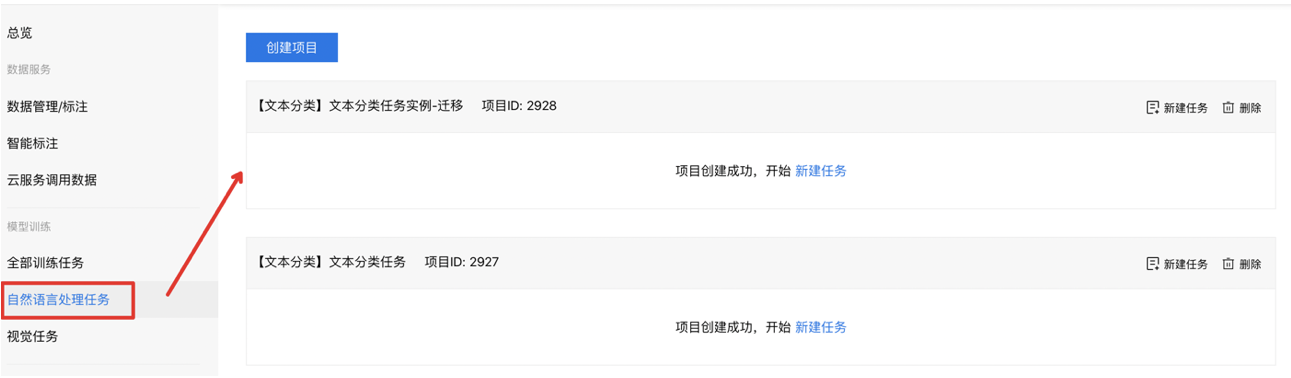# 代码模板升级及迁移说明

## 一、代码模板升级整体说明

针对平台升级新版本模型代码框架，请您关注如下几个时间点：

1. 平台于4月16日，更新上线新版代码框架，暂时保留对旧版任务的克隆功能
2. 平台于5月1日，将不再提供旧版本代码模板的克隆。当您对旧版代码模板克隆任务时，平台仅提供新版代码框架，请您做好代码的迁移工作。

说明：您在5月1日前，基于旧版本代码模板发布的任务，可长期在线上进行云端调用或本地使用。

后续平台也将基于升级后框架，继续新增任务类型，您可以加入EasyDL专业版用户交流群，针对您在模型开发过程中的需要，提出您所需的功能需求。

## 二、如何操作迁移到新版任务下

将老版本任务迁移到新版本的任务，以分类任务为例可分为三步：

第一步：查看老版本的任务中，代码编辑部分，存在哪些字段的修改

在任务列表中，找到对应的任务详情：在代码配置详情中，找到脚本内容部分，查看修改的代码内容：第二步：在平台「自然语言处理」任务列表下，新建项目并新建任务，此任务将会是基于最新的代码模板创建第三部：在下图中代码编辑部分，将第一步中对应修改过的代码部分，在新任务中进行逐一调整（关于新版代码模板的说明，详见下文）## 三、新老版本的代码编辑模板区别说明

您可以通过了解旧版本的代码模板和新版本的代码模板的代码结构，来了解两版代码的编写逻辑，从而方便您将旧版本的代码迁移到新版本的代码模板中。我们将以文本分类任务中，使用ERNIE和BOW网络的设计进行讲解说明。

下面我们先看一下旧版本的代码编辑模板的内容：

### 3.1 旧版代码模板说明

#### 1. 模型训练的配置信息

关于模型训练过程中，关于学习率、batch size等配置，代码如下：

``````def __init__(self):
Config.__init__(self)
self.batch_size = 8  # batch_size大小,尽量设置成2的整数倍
self.learning_rate = 2e-5  # 学习率设置
self.save_steps = 500  # 模型保存的间隔，即训练多少个batch之后保存一次模型，请设置成100的倍数
self.epoch = 3  # 训练多少轮
self.skip_steps = 10  # 间隔多少个batch时打印训练日志``````

组网部分，包括了ERNIE表示、BOW模型表示和分类任务几个部分，代码介绍如下：

#### 2. 网络设计中ERNIE表示的代码部分

`` emb = fluid.layers.sequence_unpad(ernie_output["sequence_output"], length=ernie_input["seq_lens"])``

#### 3. 网络设计中BOW的模型表示代码部分

``````  # bow layer
bow = fluid.layers.sequence_pool(input=emb, pool_type='sum')
bow_tanh = fluid.layers.tanh(bow)
# full connect layer
fc_1 = fluid.layers.fc(input=bow_tanh, size=hid_dim, act="tanh")
fc_2 = fluid.layers.fc(input=fc_1, size=hid_dim2, act="tanh")``````

#### 4. 网络设计中分类器的代码部分

`` logits = fluid.layers.fc(input=fc_2, size=cfg.num_labels)``

#### 5. 网络设计中预测部分的内容

`````` if is_inference:
probs = fluid.layers.softmax(logits)
feed_targets_name = [
ernie_input["src_ids"].name, ernie_input["sent_ids"].name,
]
return feed_targets_name, probs``````

#### 6. 网络设计中评估部分的代码

``````ce_loss, probs = fluid.layers.softmax_with_cross_entropy(
logits=logits, label=label, return_softmax=True)
loss = fluid.layers.mean(x=ce_loss)
graph_vars = {
"loss": loss,
"classify_infer": probs,
"label": label
}``````

### 3.2 新版代码模板说明

下文中，我们将介绍新升级的代码模板结构，您可以对比新旧版本对应的结构来进行代码的迁移：

#### 1. 新版本代码结构：模型训练的配置信息

``````TrainerConfig = {
"batch_size": 8,
"learning_rate": 2e-05,
"epoch": 3,
"train_log_step": 10,
"save_model_step": 500
}``````

#### 2. 新版本代码结构：数据读入的部分

`````` fields_dict = self.fields_process(fields_dict, phase)
instance_text_a = fields_dict["text_a"]
record_id_text_a = instance_text_a[InstanceName.RECORD_ID]
text_a_src = record_id_text_a[InstanceName.SRC_IDS]
text_a_pos = record_id_text_a[InstanceName.POS_IDS]
text_a_sent = record_id_text_a[InstanceName.SENTENCE_IDS]
text_a_lens = record_id_text_a[InstanceName.SEQ_LENS]
instance_label = fields_dict["label"]
record_id_label = instance_label[InstanceName.RECORD_ID]
label = record_id_label[InstanceName.SRC_IDS]``````

#### 3. 新版本代码结构：读取ERNIE表示

``````
emb_dict = self.make_embedding(fields_dict, phase)
emb = emb_dict["text_a"]
``````

#### 4. 新版本代码：模型超参数的设置

``````        hid_dim = 128
hid_dim2 = 96
num_labels = 2``````

#### 5. 新版本代码：BOW模型表示部分

``````   # bow layer
bow = fluid.layers.sequence_pool(input=text_a_emb, pool_type='sum')
bow_tanh = fluid.layers.tanh(bow)``````

#### 6. 新版本代码：分类器部分的代码

``````        fc_1 = fluid.layers.fc(input=bow_tanh, size=hid_dim, act="tanh")
fc_2 = fluid.layers.fc(input=fc_1, size=hid_dim2, act="tanh")
logits = fluid.layers.fc(input=fc_2, size=num_labels)``````

#### 7. 新版本代码：预测部分的内容

``````if phase == InstanceName.SAVE_INFERENCE:
"""保存模型时需要的入参：表示预测时最终输出的结果"""
probs = fluid.layers.softmax(logits)
target_predict_list = [probs]
target_feed_name_list = [text_a_src.name, text_a_pos.name, text_a_sent.name,
emb_params = self.model_params.get("embedding")
ernie_config = ErnieConfig(emb_params.get("config_path"))

forward_return_dict = {
InstanceName.TARGET_FEED_NAMES: target_feed_name_list,
InstanceName.TARGET_PREDICTS: target_predict_list
}
return forward_return_dict``````

#### 8. 新版本代码：loss部分的设置

``````   cost, probs = fluid.layers.softmax_with_cross_entropy(
logits=logits, label=label, return_softmax=True)
avg_cost = fluid.layers.mean(x=cost)
"""PREDICT_RESULT,LABEL,LOSS 是关键字，必须要赋值并返回"""``````
上一篇
创建NLP项目和任务
下一篇
NLP模型部署